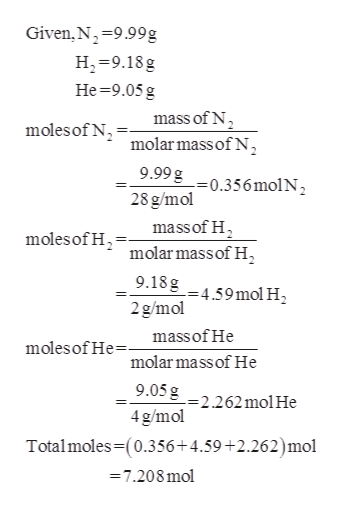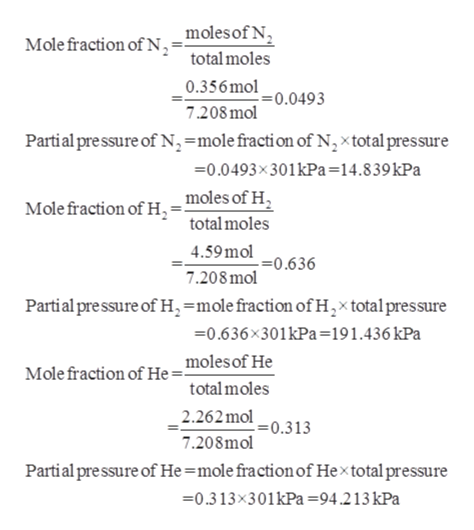# I mix 9.99 grams of nitrogen, 9.18 grams of hydrogen and 9.05 grams of helium in a flask. If the total pressure in the system was found to be 301 kPa. How much pressure of the total does each gas exert?a) The pressure due to the hydrogen was  b) The pressure due to the nitrogen was c) The pressure due to the helium was

Question

I mix 9.99 grams of nitrogen, 9.18 grams of hydrogen and 9.05 grams of helium in a flask. If the total pressure in the system was found to be 301 kPa. How much pressure of the total does each gas exert?

a) The pressure due to the hydrogen was

b) The pressure due to the nitrogen was

c) The pressure due to the helium was

check_circleExpert Solution
Step 1

From the given masses of nitrogen, hydrogen and helium, the moles can be calculated.help_outlineImage TranscriptioncloseGiven, N 9.99g H-9.18g Не 39.05 g mass of N moles of N molar mass of N 9.99g = 0.356 mol N 2 28 g/mol mass of H2 molar mass of H moles of H 9.18g =4.59 mol H2 2g/mol massof He molesof He molar mass of He 9.05g =2.262molHe 4 g/mol Totalmoles-(0.356+4.59 +2.262)mol 7.208 mol fullscreen
Step 2

From the given total pressure, the pressure ex...help_outlineImage Transcriptionclose_ moles of N Mole fraction of N total moles 0.356 mol =0.0493 7.208mol Partial pre ssure of Nn,=molefracti on of N,xtotal pressure =0.0493x301kPa=14.8 39 kPa moles of H Mole fraction of H total moles 4.59mol =0.636 7.208mol Partial pre ssure of H=mole fraction of H,x total pressure 0.636x301kPa=191.436 kPa moles of He Mole fraction of He= total moles 2.262mol 7.208mol .=0.3 13 Partial pressure of He =mole fraction of Hextotal pressure 0.313x301kPa =94.213 kPa fullscreen

### Want to see the full answer?

See Solution

#### Want to see this answer and more?

Solutions are written by subject experts who are available 24/7. Questions are typically answered within 1 hour*

See Solution
*Response times may vary by subject and question
Tagged in

### Physical Chemistry